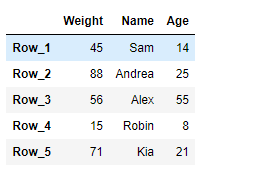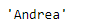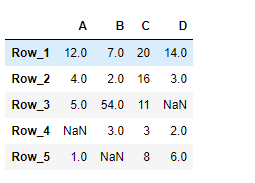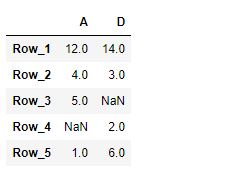Related Articles

# Python | Pandas DataFrame.loc[]

• Difficulty Level : Basic
• Last Updated : 20 Feb, 2019

Pandas DataFrame is a two-dimensional size-mutable, potentially heterogeneous tabular data structure with labeled axes (rows and columns). Arithmetic operations align on both row and column labels. It can be thought of as a dict-like container for Series objects. This is the primary data structure of the Pandas.

Pandas` DataFrame.loc` attribute access a group of rows and columns by label(s) or a boolean array in the given DataFrame.

Syntax: DataFrame.loc

Parameter : None

Returns : Scalar, Series, DataFrame

Example #1: Use `DataFrame.loc` attribute to access a particular cell in the given Dataframe using the index and column labels.

 `# importing pandas as pd``import` `pandas as pd`` ` `# Creating the DataFrame``df ``=` `pd.DataFrame({``'Weight'``:[``45``, ``88``, ``56``, ``15``, ``71``],``                   ``'Name'``:[``'Sam'``, ``'Andrea'``, ``'Alex'``, ``'Robin'``, ``'Kia'``],``                   ``'Age'``:[``14``, ``25``, ``55``, ``8``, ``21``]})`` ` `# Create the index``index_ ``=` `[``'Row_1'``, ``'Row_2'``, ``'Row_3'``, ``'Row_4'``, ``'Row_5'``]`` ` `# Set the index``df.index ``=` `index_`` ` `# Print the DataFrame``print``(df)`

Output :Now we will use `DataFrame.loc` attribute to return the value present in the ‘Name’ column corresponding to the ‘Row_2’ label.

 `# return the value``result ``=` `df.loc[``'Row_2'``, ``'Name'``]`` ` `# Print the result``print``(result)`

Output :As we can see in the output, the `DataFrame.loc` attribute has successfully returned the value present at the desired location in the given DataFrame.

Example #2: Use `DataFrame.loc` attribute to return two of the column in the given Dataframe.

 `# importing pandas as pd``import` `pandas as pd`` ` `# Creating the DataFrame``df ``=` `pd.DataFrame({``"A"``:[``12``, ``4``, ``5``, ``None``, ``1``], ``                   ``"B"``:[``7``, ``2``, ``54``, ``3``, ``None``], ``                   ``"C"``:[``20``, ``16``, ``11``, ``3``, ``8``], ``                   ``"D"``:[``14``, ``3``, ``None``, ``2``, ``6``]}) `` ` `# Create the index``index_ ``=` `[``'Row_1'``, ``'Row_2'``, ``'Row_3'``, ``'Row_4'``, ``'Row_5'``]`` ` `# Set the index``df.index ``=` `index_`` ` `# Print the DataFrame``print``(df)`

Output :Now we will use `DataFrame.loc` attribute to return the values present in the ‘A’ and ‘D’ column of the Dataframe.

 `# return the values.``result ``=` `df.loc[:, [``'A'``, ``'D'``]]`` ` `# Print the result``print``(result)`

Output :As we can see in the output, the `DataFrame.loc` attribute has successfully returned the desired columns of the dataframe.

Attention geek! Strengthen your foundations with the Python Programming Foundation Course and learn the basics.

To begin with, your interview preparations Enhance your Data Structures concepts with the Python DS Course. And to begin with your Machine Learning Journey, join the Machine Learning – Basic Level Course

My Personal Notes arrow_drop_up